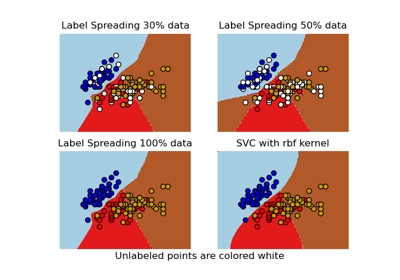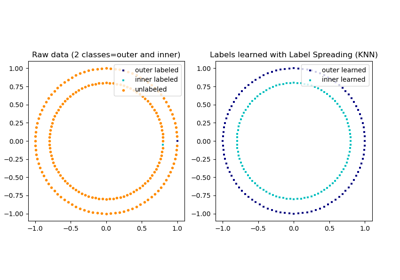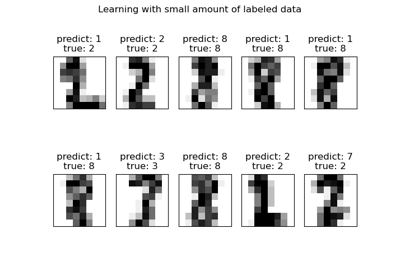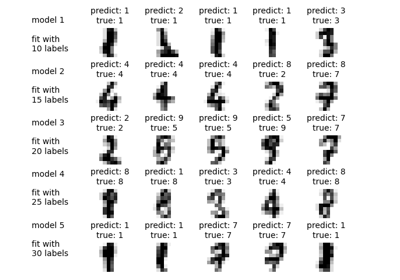# sklearn.semi_supervised.LabelSpreading¶

class sklearn.semi_supervised.LabelSpreading(kernel='rbf', *, gamma=20, n_neighbors=7, alpha=0.2, max_iter=30, tol=0.001, n_jobs=None)[source]

This model is similar to the basic Label Propagation algorithm, but uses affinity matrix based on the normalized graph Laplacian and soft clamping across the labels.

Read more in the User Guide.

Parameters
kernel{‘knn’, ‘rbf’} or callable, default=’rbf’

String identifier for kernel function to use or the kernel function itself. Only ‘rbf’ and ‘knn’ strings are valid inputs. The function passed should take two inputs, each of shape (n_samples, n_features), and return a (n_samples, n_samples) shaped weight matrix.

gammafloat, default=20

Parameter for rbf kernel.

n_neighborsint, default=7

Parameter for knn kernel which is a strictly positive integer.

alphafloat, default=0.2

Clamping factor. A value in (0, 1) that specifies the relative amount that an instance should adopt the information from its neighbors as opposed to its initial label. alpha=0 means keeping the initial label information; alpha=1 means replacing all initial information.

max_iterint, default=30

Maximum number of iterations allowed.

tolfloat, default=1e-3

Convergence tolerance: threshold to consider the system at steady state.

n_jobsint, default=None

The number of parallel jobs to run. None means 1 unless in a joblib.parallel_backend context. -1 means using all processors. See Glossary for more details.

Attributes
X_ndarray of shape (n_samples, n_features)

Input array.

classes_ndarray of shape (n_classes,)

The distinct labels used in classifying instances.

label_distributions_ndarray of shape (n_samples, n_classes)

Categorical distribution for each item.

transduction_ndarray of shape (n_samples,)

Label assigned to each item via the transduction.

n_iter_int

Number of iterations run.

LabelPropagation

Unregularized graph based semi-supervised learning

References

Dengyong Zhou, Olivier Bousquet, Thomas Navin Lal, Jason Weston, Bernhard Schoelkopf. Learning with local and global consistency (2004) http://citeseer.ist.psu.edu/viewdoc/summary?doi=10.1.1.115.3219

Examples

>>> import numpy as np
>>> from sklearn import datasets
>>> rng = np.random.RandomState(42)
>>> random_unlabeled_points = rng.rand(len(iris.target)) < 0.3
>>> labels = np.copy(iris.target)
>>> labels[random_unlabeled_points] = -1
>>> label_prop_model.fit(iris.data, labels)


Methods

 fit(X, y) Fit a semi-supervised label propagation model based get_params([deep]) Get parameters for this estimator. Performs inductive inference across the model. Predict probability for each possible outcome. score(X, y[, sample_weight]) Return the mean accuracy on the given test data and labels. set_params(**params) Set the parameters of this estimator.
__init__(kernel='rbf', *, gamma=20, n_neighbors=7, alpha=0.2, max_iter=30, tol=0.001, n_jobs=None)[source]

Initialize self. See help(type(self)) for accurate signature.

fit(X, y)[source]

Fit a semi-supervised label propagation model based

All the input data is provided matrix X (labeled and unlabeled) and corresponding label matrix y with a dedicated marker value for unlabeled samples.

Parameters
Xarray-like of shape (n_samples, n_features)

A matrix of shape (n_samples, n_samples) will be created from this.

yarray-like of shape (n_samples,)

n_labeled_samples (unlabeled points are marked as -1) All unlabeled samples will be transductively assigned labels.

Returns
selfobject
get_params(deep=True)[source]

Get parameters for this estimator.

Parameters
deepbool, default=True

If True, will return the parameters for this estimator and contained subobjects that are estimators.

Returns
paramsmapping of string to any

Parameter names mapped to their values.

predict(X)[source]

Performs inductive inference across the model.

Parameters
Xarray-like of shape (n_samples, n_features)

The data matrix.

Returns
yndarray of shape (n_samples,)

Predictions for input data.

predict_proba(X)[source]

Predict probability for each possible outcome.

Compute the probability estimates for each single sample in X and each possible outcome seen during training (categorical distribution).

Parameters
Xarray-like of shape (n_samples, n_features)

The data matrix.

Returns
probabilitiesndarray of shape (n_samples, n_classes)

Normalized probability distributions across class labels.

score(X, y, sample_weight=None)[source]

Return the mean accuracy on the given test data and labels.

In multi-label classification, this is the subset accuracy which is a harsh metric since you require for each sample that each label set be correctly predicted.

Parameters
Xarray-like of shape (n_samples, n_features)

Test samples.

yarray-like of shape (n_samples,) or (n_samples, n_outputs)

True labels for X.

sample_weightarray-like of shape (n_samples,), default=None

Sample weights.

Returns
scorefloat

Mean accuracy of self.predict(X) wrt. y.

set_params(**params)[source]

Set the parameters of this estimator.

The method works on simple estimators as well as on nested objects (such as pipelines). The latter have parameters of the form <component>__<parameter> so that it’s possible to update each component of a nested object.

Parameters
**paramsdict

Estimator parameters.

Returns
selfobject

Estimator instance.

## Examples using sklearn.semi_supervised.LabelSpreading¶